• 内容大部分摘自下《漫画算法 小灰的算法之旅》，加了自己的一部分想法 数组：随机读取，顺序存储 1. 读取数据 int array = {1,2,3,4,5} array[index] 2. 更新元素 int array = {1,2,3,4,5} ar...
目录数组：随机读取，顺序存储1. 读取数据2. 更新元素3. 插入元素3.1. 尾部插入3.2. 中间插入3.3. 超范围输入4. 删除元素Java实现可扩展数组完整代码（插入+删除）

大家好，我是爱做梦的鱼，我是一个大三的小菜鸡，非常向往优秀，羡慕优秀的人，已拿两个暑假offer，欢迎大家找我进行交流😂😂😂
这是我的博客地址：子浩的博客https://blog.csdn.net/weixin_43124279

专栏《两周干掉数据结构》

内容大部分摘自下《漫画算法 小灰的算法之旅》，加了自己的一部分想法
System.arraycopy()会使我们对数组的操作变简单，建议去看一下以下这篇文章
java的数组复制方法System.arraycopy()的使用说明

数组：随机读取，顺序存储
1. 读取数据
int array = {1,2,3,4,5}
array[index]

2. 更新元素
int array = {1,2,3,4,5}
array[index]=newValue

数组读取元素和更新元素的时间复杂度都是O(1) 。
3. 插入元素
==在介绍插入数组元素的操作之前，我们需要补充一个概念，那就是数组
的实际元素数量有可能小于数组的长度，这里很重要。==例如下面的情形。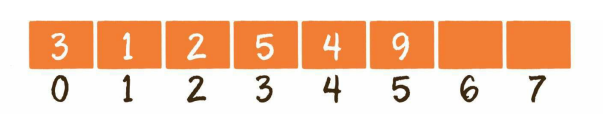因此，插入数组元素的操作存在3种情况。

尾部插入
中间插入
超范围插入

3.1. 尾部插入
尾部插入，是最简单的情况，直接把插入的元素放在数组尾部的空闲位置即可，等同于更新元素的操作。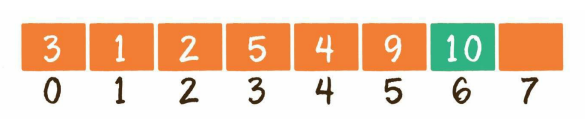public void insert(int index, int element) {
array[index] = element;
size++;
}

3.2. 中间插入
中间插入，稍微复杂一些。由于数组的每一个元素都有其固定下标，所以不得不首先把插入位置及后面的元素向后移动，腾出地方，再把要插入的元素放到对应的数组位置上。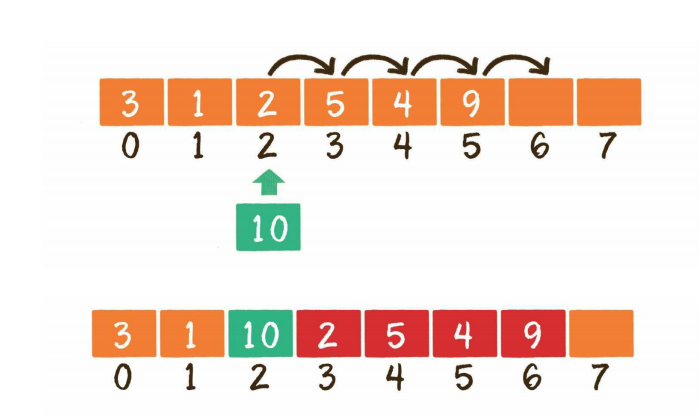/**
* Created by IntelliJ IDEA.
*
* @Author: 张志浩  Zhang Zhihao
* @Email: 3382885270@qq.com
* @Date: 2020/4/30
* @Time: 19:20
* @Version: 1.0
*/
public class MyArray3 {
private static int aa;
private int[] array;
private int size;

public MyArray3(int capacity) {
this.array = new int[capacity];
size = 0;
}

public static void main(String[] args) throws Exception {
MyArray3 myArray3 = new MyArray3(10);
// myArray2.insert(-1, 8); //超出数组实际元素范围！
// myArray2.insert(3, 8); //超出数组实际元素范围！
myArray3.insert(0, 3);
myArray3.insert(1, 7);
myArray3.insert(2, 9);
myArray3.insert(3, 5);
myArray3.insert(1, 6);
myArray3.output();
}

/**
* 数组插入元素
*
* @param index   插入的位置
* @param element 插入的元素
*/
public void insert(int index, int element) {
//判断访问下标是否超出范围
if (index < 0 || index > size) {
throw new IndexOutOfBoundsException("超出数组实际元素范围！");
}

//从右向左循环，逐个元素向右挪一位。
for (int i = size - 1; i >= index; i--) {
array[i + 1] = array[i];
}
//        System.arraycopy(array, index, array, index + 1, size - index);

//腾出的位置放入新元素
array[index] = element;
size++;
}

/**
* 输出数组
*/
public void output() {
for (int i = 0; i < size; i++) {
System.out.print(array[i] + " ");
}
}
}

该代码不只是中间插入，其实考虑了尾部插入+中间插入（头部插入算中间插入）
代码中的成员变量size是数组实际元素的数量。

如果插入元素在数组尾部，传入的下标参数index等于size；
如果插入元素在数组中间或头部，则index小于size。
如果传入的下标参数index大于size或小于0，则认为是非法输入，会直接抛出异常。

3.3. 超范围输入
假如现在有一个长度为6的数组，已经装满了元素，这时还想插入一个新元素。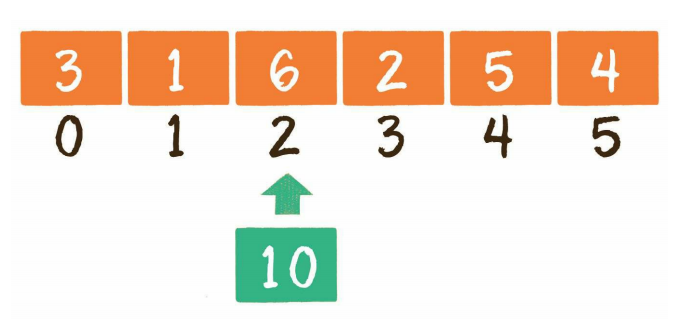这就涉及数组的扩容了。可是数组的长度在创建时就已经确定了，无法像孙悟空的金箍棒那样随意变长或变短。这该如何是好呢？此时可以创建一个新数组，长度是旧数组的2倍，再把旧数组中的元素统统复制过去，这样就实现了数组的扩容。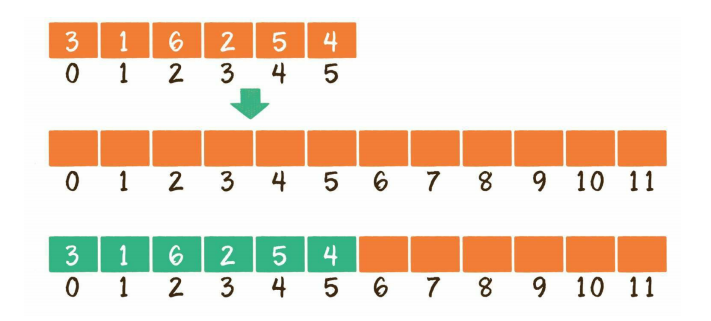/**
* Created by IntelliJ IDEA.
*
* @Author: 张志浩  Zhang Zhihao
* @Email: 3382885270@qq.com
* @Date: 2020/4/30
* @Time: 19:28
* @Version: 1.0
*/
public class MyArray4 {
private int[] array;
private int size;

public MyArray4(int capacity) {
this.array = new int[capacity];
size = 0;
}

public static void main(String[] args) throws Exception {
MyArray4 myArray4 = new MyArray4(4);
myArray4.insert(-1, 8); //超出数组实际元素范围！
myArray4.insert(3, 8); //超出数组实际元素范围！
myArray4.insert(0, 3);
myArray4.insert(1, 7);
myArray4.insert(2, 9);
myArray4.insert(3, 5);
myArray4.insert(1, 6);
myArray4.insert(5, 8); //超出数组长度4
myArray4.output();
}

/**
* 数组插入元素
*
* @param index   插入的位置
* @param element 插入的元素
*/
public void insert(int index, int element) {
//判断访问下标是否超出范围
if (index < 0 || index > size) {
throw new IndexOutOfBoundsException("超出数组实际元素范围！");
}

//如果实际元素达到数组容量上线，数组扩容
if (size >= array.length) {
resize();
}

//从右向左循环，逐个元素向右挪一位。
for (int i = size - 1; i >= index; i--) {
array[i + 1] = array[i];
}
//        System.arraycopy(array, index, array, index + 1, size - index);

//腾出的位置放入新元素
array[index] = element;
size++;
}

/**
* 数组扩容
*/
public void resize() {
int[] arrayNew = new int[array.length * 2];

//从旧数组拷贝到新数组
for (int i = 0; i < size; i++) {
arrayNew[i] = array[i];
}
//        System.arraycopy(array, 0, arrayNew, 0, array.length);

array = arrayNew;
}

/**
* 输出数组
*/
public void output() {
for (int i = 0; i < size; i++) {
System.out.print(array[i] + " ");
}
}
}

4. 删除元素
数组的删除操作和插入操作的过程相反，如果删除的元素位于数组中间，其后的元素都需要向前挪动1位。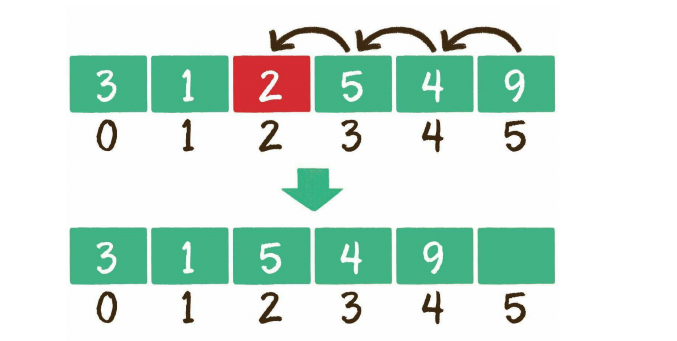/**
* 数组删除元素
*
* @param index 删除的位置
*/
public int delete(int index) throws Exception {
//判断访问下标是否超出范围
if (index < 0 || index >= size) {
throw new IndexOutOfBoundsException("超出数组实际元素范围！");
}
int deletedElement = array[index];

//从左向右循环，逐个元素向左挪一位。
for (int i = index; i < size - 1; i++) {
array[i] = array[i + 1];
}
//        System.arraycopy(array, index + 1, array, index, size - 1 - index);

size--;
return deletedElement;
}

Java实现可扩展数组完整代码（插入+删除）
有同学私聊我想要能运行的插入加删除的完整代码
/**
* Created by IntelliJ IDEA.
*
* @Author: 张志浩  Zhang Zhihao
* @Email: 3382885270@qq.com
* @Date: 2020/4/30
* @Time: 19:28
* @Version: 1.0
*/
public class MyArray {

private int[] array;
private int size;

public MyArray(int capacity) {
this.array = new int[capacity];
size = 0;
}

public static void main(String[] args) throws Exception {
MyArray myArray = new MyArray(4);
myArray.insert(0, 3);
myArray.insert(1, 7);
myArray.insert(2, 9);
myArray.insert(3, 5);
myArray.insert(1, 6);
myArray.insert(5, 8);
myArray.delete(3);
myArray.output();
}

/**
* 数组插入元素
*
* @param index   插入的位置
* @param element 插入的元素
*/
public void insert(int index, int element) throws Exception {
//判断访问下标是否超出范围
if (index < 0 || index > size) {
throw new IndexOutOfBoundsException("超出数组实际元素范围！");
}

//如果实际元素达到数组容量上线，数组扩容
if (size >= array.length) {
resize();
}

//从右向左循环，逐个元素向右挪一位。
for (int i = size - 1; i >= index; i--) {
array[i + 1] = array[i];
}
//        System.arraycopy(array, index, array, index + 1, size - index);

//腾出的位置放入新元素
array[index] = element;
size++;
}

/**
* 数组扩容
*/
public void resize() {
int[] arrayNew = new int[array.length * 2];

//从旧数组拷贝到新数组
for (int i = 0; i < size; i++) {
arrayNew[i] = array[i];
}
//        System.arraycopy(array, 0, arrayNew, 0, array.length);

array = arrayNew;
}

/**
* 数组删除元素
*
* @param index 删除的位置
*/
public int delete(int index) throws Exception {
//判断访问下标是否超出范围
if (index < 0 || index >= size) {
throw new IndexOutOfBoundsException("超出数组实际元素范围！");
}
int deletedElement = array[index];

//从左向右循环，逐个元素向左挪一位。
for (int i = index; i < size - 1; i++) {
array[i] = array[i + 1];
}
//        System.arraycopy(array, index + 1, array, index, size - 1 - index);

size--;
return deletedElement;
}

/**
* 输出数组
*/
public void output() {
for (int i = 0; i < size; i++) {
System.out.print(array[i] + " ");
}
}
}



展开全文算法 java array
• /* (程序头部注释开始) * 程序的版权和版本声明部分 * Copyright (c) 2011, 烟台大学计算机学院学生 ... * All rights reserved. * 文件名称： 《数组——数据结构JAVA》  * 作 者： 刘江波  * 完成日期： 2
/* (程序头部注释开始)
* 程序的版权和版本声明部分
* 文件名称：    《数组——数据结构JAVA》
* 作    者：       刘江波
* 完成日期：    2012     年    10   月     14   日
* 版 本 号：    V2.1
* 对任务及求解方法的描述部分
* 问题描述：
* 程序头部的注释结束
*/
public class Array {
private int[]Array;
private int ArraySize;
private int ArrayLength;
private void GetArray(){
//私有函数，动态分配数组存储空间
Array = new int[ArraySize];
if(Array == null)
System.out.println("Memory Allocation Error");
}
public Array(int size){
//构造函数，建立一个最大元素个数为size的数组
if(size <= 0)
System.out.println("Invalid Array Size");
else{
ArraySize = size;
ArrayLength = 0;
GetArray();
}
}
//求数组元素个数
public int GetLength(){
return ArrayLength;
}
public int GetNode(int i){
//取数组中第i个结点的值，若存在，则返回该结点的值；
//否则返回null；
return(i<0||i>ArrayLength)?null:Array[i];
}
public int Find(int x){
//查找值为x的结点，若找到，则返回结点序号，否则返回-1；
for(int i=0; i<ArrayLength; i++)
if(Array[i] == x)return i;
return -1;
}
//在数组第i个位置，插入值为x的结点，若插入成功，返回true；否则返回false；
public boolean Insert(int x,int i){
if(ArrayLength == ArraySize){
System.out.println("overflow");
return false;
}
else if(i<0 || i>ArrayLength){
System.out.println("position error");
return false;
}
else
{
for(int j=ArrayLength-1; j>=i; j--)
Array[j+1] = Array[j];//后移
Array[i] = x;         //插入
ArrayLength++;        //数组长度加一
return true;
}
}
//删除第i个元素结点，成功返回true，否则返回false;
public boolean Remove(int i){
if(ArrayLength == 0){
System.out.println("Array Is Empty");
return false;
}
else if(i<0 || i>ArrayLength-1){
System.out.println("position error");
return false;
}
else
{
for(int j=i; j<ArrayLength-1; j++)
Array[j] = Array[j+1];//前移
ArrayLength--;
return true;
}
}
//将两个数组进行“并”运算
public void Union(Array a,Array b){
//将数组b合并到a中，重复元素只留一个；
int n = a.GetLength();
int m = b.GetLength();
for(int i=0; i<m; i++){
int x = b.GetNode(i); //从b中取出一元素
int k = b.Find(x);    //在a中查找同值元素
if(k == -1){          //若找不到同值元素
a.Insert(x, n);   //则查到a的最后
n++;
}
}
}
//将两个数组进行“交”运算
public void Intersection(Array a,Array b){
//求两数组相同元素，存到b中
//int n = a.GetLength();
int m = b.GetLength();
int i = 0;
while(i<m){
int x = b.GetNode(i); //从b中取出一元素
int k = a.Find(x);    //在a中查找等值元素
if(k == -1){          //若没有找到等值元素
b.Remove(i);      //从b中删除该元素
m--;
}
else i++;             //否则，在b中保留该元素
}
}
}



展开全文java allocation insert null
• Nginx数组属于基础数据结构部分，只要具备一定的开发经验，可以参考自己所熟悉的其他框架的数组。值得重复提出的一点是Nginx数组的内存事宜遵循，Nginx内存使用的类别化，层级化的原则，内存的使用在内存池中进行。 ...
       nginx内部使用的数组结构是ngx_array_t，ngx_array_t是一个顺序容器，以数组的形式存储元素，并能够在容量达到最大值时动态扩容。Nginx数组属于基础数据结构部分，只要具备一定的开发经验，可以参考自己所熟悉的其他框架的数组。值得重复提出的一点是Nginx数组的内存事宜遵循，Nginx内存使用的类别化，层级化的原则，内存的使用在内存池中进行。

1.数据结构

typedef struct {
void        *elts; /*数据区域的起始地址指针*/
ngx_uint_t   nelts; /*已经存储的元素数量*/
size_t       size;  /*单个元素的内存大小，Nginx数组的每个元素大小都相同*/
ngx_uint_t   nalloc;/*数组已经分配的内存空间数量*/
ngx_pool_t  *pool; /*数组所在内存池地址*/
} ngx_array_t;

从数据结构看，Nginx的数组本身只包含数组的管理，不保存实际的数据内容，同时Nginx的数组内存分配也必须是基于内存池实现，这点在数组的使用过程中体现的更加明显。

2.数组操作
2.1数组初始化

数组初始化参数包括，数组指针，用来分配数组内存的内存池，数组可以存储的数据长度，每个元素占用的空间大小。
初始化时已经存储的数量为0，然后在传入内存池中分配，数据长度*数据空间大小长度的内存给数组。

static ngx_inline ngx_int_t
ngx_array_init(ngx_array_t *array, ngx_pool_t *pool, ngx_uint_t n, size_t size)
{
/*
* set "array->nelts" before "array->elts", otherwise MSVC thinks
* that "array->nelts" may be used without having been initialized
*/

array->nelts = 0;
array->size = size;
array->nalloc = n;
array->pool = pool;

array->elts = ngx_palloc(pool, n * size);
if (array->elts == NULL) {
return NGX_ERROR;
}

return NGX_OK;
}

2.2数组创建

ngx_array_t *
ngx_array_create(ngx_pool_t *p, ngx_uint_t n, size_t size)
{
ngx_array_t *a;

a = ngx_palloc(p, sizeof(ngx_array_t));
if (a == NULL) {
return NULL;
}

if (ngx_array_init(a, p, n, size) != NGX_OK) {
return NULL;
}

return a;
}

2.3添加一个元素

ngx_array_t数组添加元素主要工作是指针的移动和数据的管理，比如移动最后指针的位置，更新已存储数据nelts的值，存储空间size的值，可存储数据nalloc的值，以及在空间不足的时候扩大原来两倍的空内存空间。

void *
ngx_array_push(ngx_array_t *a)
{
void        *elt, *new;
size_t       size;
ngx_pool_t  *p;

if (a->nelts == a->nalloc) {

/* the array is full */

size = a->size * a->nalloc;

p = a->pool;

if ((u_char *) a->elts + size == p->d.last
&& p->d.last + a->size <= p->d.end)
{
/*
* the array allocation is the last in the pool
* and there is space for new allocation
*/

p->d.last += a->size;
a->nalloc++;

} else {
/* allocate a new array */

new = ngx_palloc(p, 2 * size);
if (new == NULL) {
return NULL;
}

ngx_memcpy(new, a->elts, size);
a->elts = new;
a->nalloc *= 2;
}
}

elt = (u_char *) a->elts + a->size * a->nelts;
a->nelts++;

return elt;
}

Nginx数组操作不涉及数据内容的移动只在于指针的移动速度快、数组容量可动态扩容，不足之处在于动态扩容每次都是扩容为原来的两倍，不能根据实际需要分配。

展开全文• 树状数组基础 单点更新: 区间查询: 高级操作 求逆序对 操作 原理 求区间最大值 区间修改+单点查询 查询 修改 区间修改+区间查询 查询 修改 二维树状数组 单点修改+区间查询 区间修改 + 单点查询...
目录

转载请注明出处:bestsort.cn

树状数组基础

单点更新:

区间查询:

高级操作

求逆序对

操作

原理

求区间最大值

区间修改+单点查询

查询

修改

区间修改+区间查询

查询

修改

二维树状数组

单点修改+区间查询

区间修改 + 单点查询

区间修改 + 区间查询

转载请注明出处:bestsort.cn

树状数组基础

树状数组是一个查询和修改复杂度都为log(n)的数据结构。主要用于数组的单点修改&&区间求和.

另外一个拥有类似功能的是线段树.

具体区别和联系如下：

1.两者在复杂度上同级, 但是树状数组的常数明显优于线段树, 其编程复杂度也远小于线段树.

2.树状数组的作用被线段树完全涵盖, 凡是可以使用树状数组解决的问题, 使用线段树一定可以解决, 但是线段树能够解决的问题树状数组未必能够解决.

3.树状数组的突出特点是其编程的极端简洁性, 使用lowbit技术可以在很短的几步操作中完成树状数组的核心操作，其代码效率远高于线段树。

上面出现了一个新名词:lowbit.其实lowbit(x)就是求x最低位的1;

下面加图进行解释

对于一般的二叉树,我们是这样画的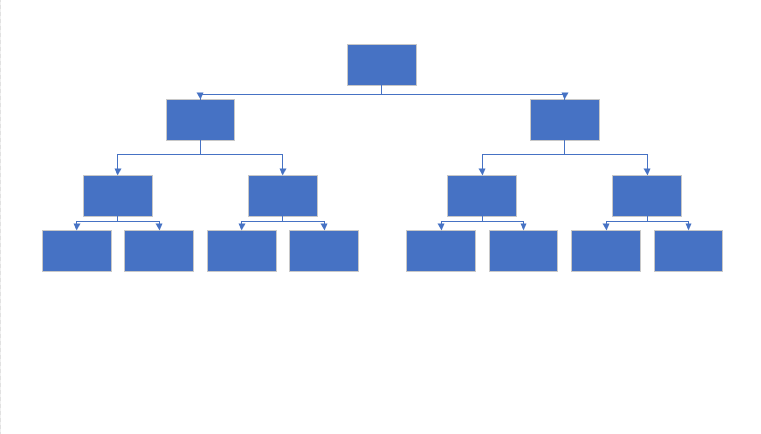把位置稍微移动一下,便是树状数组的画法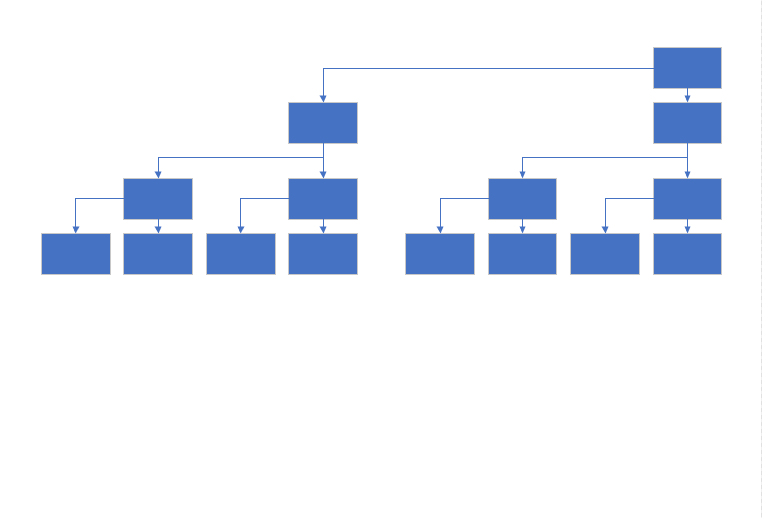一图秒懂

重头戏来了，bestsort教你一图学会树状数组~(咱也不知道为啥其他博客写那么复杂

需要注意的是，图中的子节点包括自己，比如说8这个节点，里面的值是原始数组中[5,8]的和

标记为灰色的节点实际已被上层覆盖,不占据空间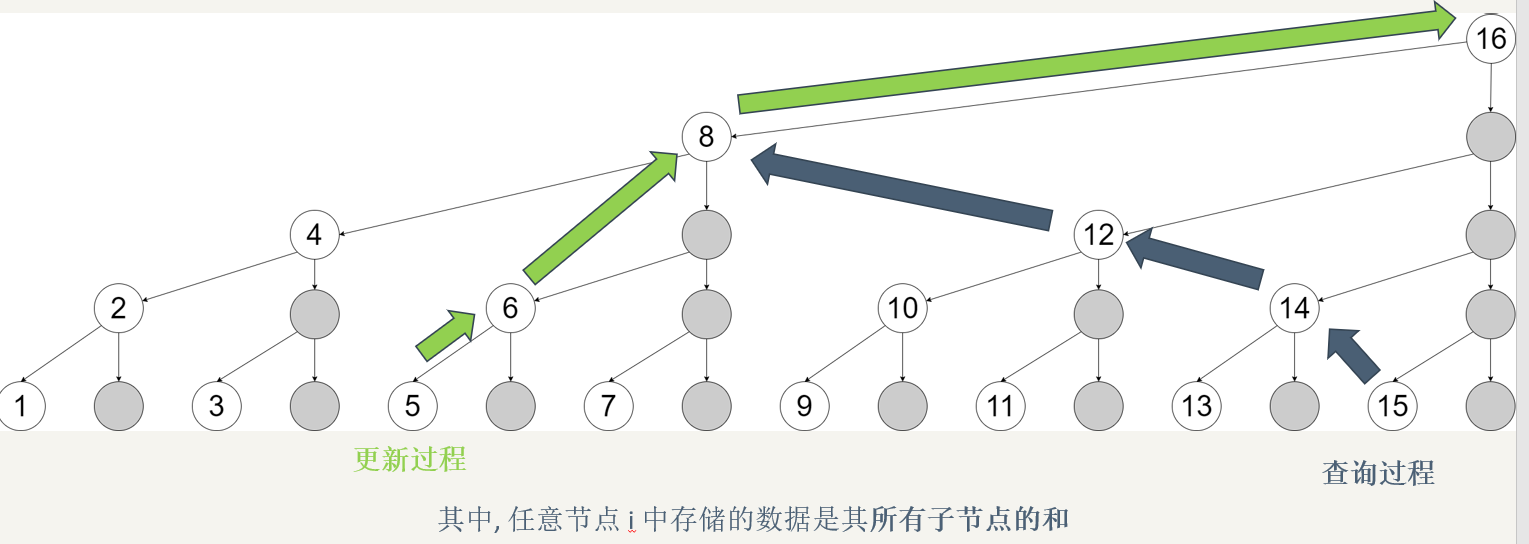下面是二进制版本，能看到

更新过程是每次加了个二进制的低位1(101+1 ->110, 110 + 10 -> 1000, 1000 + 1000 -> 10000)

查询过程每次就是去掉了二进制中的低位1(1111 - 1 -> 1110, 1110 - 10 -> 1100, 1100 - 100 -> 1000)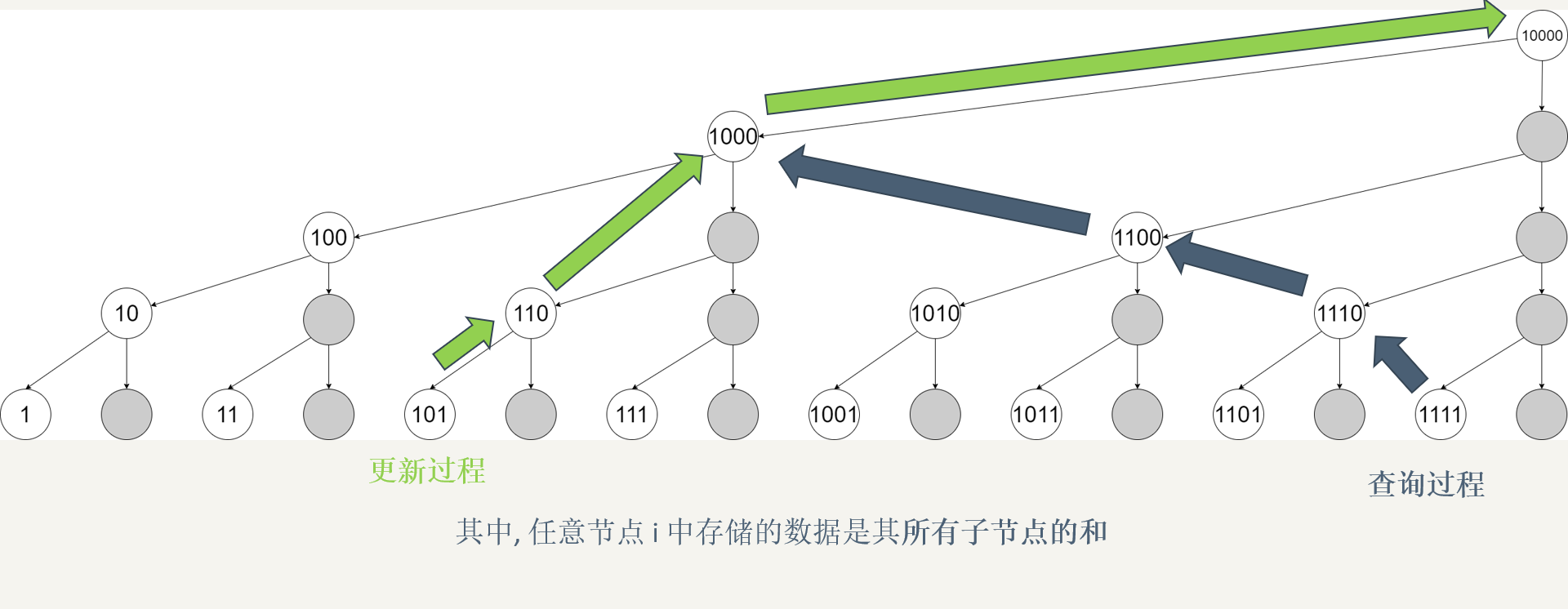开篇就说了,lowbit(x)是取出x的最低位1;具体操作为

int lowbit(x){return x&(-x);}

极致简短!!!!现在我们来理解一下这行代码:

我们知道,对于一个数的负数就等于对这个数取反+1

以二进制数11010为例:11010的补码为00101,加1后为00110,两者相与便是最低位的1

其实很好理解,补码和原码必然相反,所以原码有0的部位补码全是1,补码再+1之后由于进位那么最末尾的1和原码

最右边的1一定是同一个位置(当遇到第一个1的时候补码此位为0,由于前面会进一位,所以此位会变为1)

所以我们只需要进行a&(-a)就可以取出最低位的1了

会了lowbit,我们就可以进行区间查询和单点更新了!!!

--------------------------------------------------------------------------------------------

单点更新:

继续看开始给出的图

此时如果我们要更改A

则有以下需要进行同步更新

1(001)        C+=A

lowbit(1)=001 1+lowbit(1)=2(010)     C+=A

lowbit(2)=010 2+lowbit(2)=4(100)     C+=A

lowbit(4)=100 4+lowbit(4)=8(1000)   C+=A

换成代码就是:

void update(int x,int y,int n){
for(int i=x;i<=n;i+=lowbit(i))    //x为更新的位置,y为更新后的数,n为数组最大值
c[i] += y;
}

--------------------------------------------------------------------------------------------

区间查询:

举个例子 i=5

C=A+A+A+A;

C=A;

可以推出:   sum(i = 5)  ==> C+C;

序号写为二进制: sum(101)=C[(100)]+C[(101)];

第一次101,减去最低位的1就是100;

其实也就是单点更新的逆操作

代码如下:

int getsum(int x){
int ans = 0;
for(int i=x;i;i-=lowbit(i))
ans += c[i];
return ans;
}

lowbit会了,区间查询有了,单点更新也有了接下来该做题了

单击传送门移步HDU1166 敌兵布阵

附代码:

#include <iostream>
#include <cstdio>
#include <cstdlib>
#include <cstring>
#include <cmath>
#include <algorithm>
#include <queue>
#include <string>
#include <vector>
#define For(a,b) for(int a=0;a<b;a++)
#define mem(a,b) memset(a,b,sizeof(a))
#define _mem(a,b) memset(a,0,(b+1)<<2)
#define lowbit(a) ((a)&-(a))
using namespace std;
typedef long long ll;
const int maxn =  5*1e4+5;
const int INF = 0x3f3f3f3f;
int c[maxn];
void update(int x,int y,int n){
for(int i=x;i<=n;i+=lowbit(i))
c[i] += y;
}
int getsum(int x){
int ans = 0;
for(int i=x;i;i-=lowbit(i))
ans += c[i];
return ans;
}
int main()
{
int t;
int n;
int x,y,z;
string s;
cin >> t ;
for(int j=1;j<=t;j++){
scanf("%d",&n);
_mem(c,n);      //初始化数组中前n+1个数为0
for(int i=1;i<=n;i++){
scanf("%d",&z);
update(i,z,n);
}
cout <<"Case "<<j<<":"<<endl;
while(1){
cin >> s;
if(s == 'E')
break;
scanf("%d%d",&x,&y);
if(s == 'Q')
cout << getsum(y)-getsum(x-1)<<endl;
else if(s == 'A')
update(x,y,n);
else
update(x,-y,n);
}
}
return 0;
}


高级操作

求逆序对

操作

对于数组a，我们将其离散化处理为b[].区间查询与单点修改代码如下

void update(int p)
{
while(p<=n)
{
a[p] ++;
p+=lowbit(p);
}
}

int getsum(int p)
{
int res = 0;
while(p)
res += a[p],p -= lowbit(p);
return res;
}


a的逆序对个数为：

for(int i=1;i<=n;i++){
update(b[i]+1);
res += i-getsum(b[i]+1);
}

原理

此部分来自ssimple_y的博客

第一次插入的时候把5这个位置上加上1，read(x)值就是1，当前已经插入了一个数，所以他前面比他大的数的个数就等于 i - read(x) = 1 - 1 = 0，所以总数 sum += 0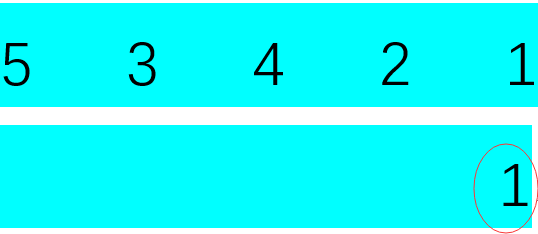第二次插入的时候，read(x)的值同样是1，但是 i - read(x) = 2 - 1 = 1，所以sum += 1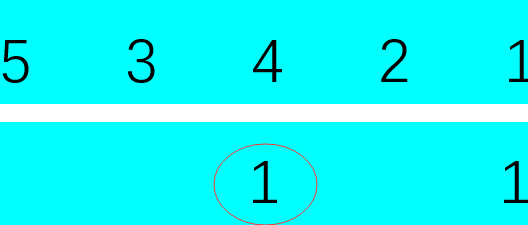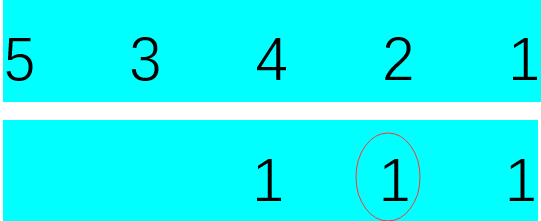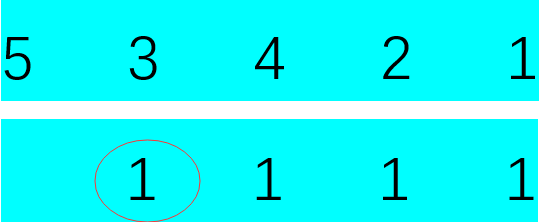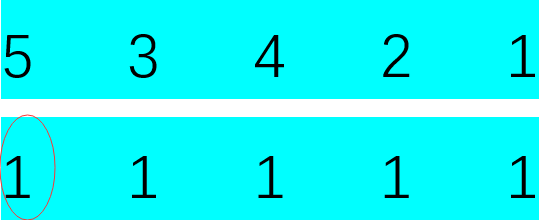这样整个过程就结束了，所有的逆序对就求出来了。

求区间最大值

void Update(int i,int v)
{
while(i<=maxY)
{
t[i] = max(t[i],v);
i += lowbit(i);
}
}

int query(int i)
{
int ans = 0;
while(i)
{
ans = max(ans,t[i]);
i -= lowbit(i);
}
return ans;
}

该部分内容转自胡小兔的OI博

区间修改+单点查询

通过“差分”（就是记录数组中每个元素与前一个元素的差），可以把这个问题转化为问题1。

查询

设原数组为, 设数组，则，可以通过求的前缀和查询。

修改

当给区间加上x的时候，与前一个元素 的差增加了x，与 的差减少了x。根据数组的定义，只需给加上 x, 给 减去x即可

void add(int p, int x){ //这个函数用来在树状数组中直接修改
while(p <= n) sum[p] += x, p += p & -p;
}
void range_add(int l, int r, int x){ //给区间[l, r]加上x
}
int res = 0;
while(p) res += sum[p], p -= p & -p;
return res;
}

区间修改+区间查询

这是最常用的部分，也是用线段树写着最麻烦的部分——但是现在我们有了树状数组！

怎么求呢？我们基于问题2的“差分”思路，考虑一下如何在问题2构建的树状数组中求前缀和：

位置p的前缀和 =

在等式最右侧的式子中，被用了p次，被用了次……那么我们可以写出：

位置p的前缀和 =

那么我们可以维护两个数组的前缀和：
一个数组是
另一个数组是

查询

位置p的前缀和即：数组中p的前缀和 - sum2数组中p的前缀和。

区间[l, r]的和即：位置r的前缀和 - 位置l的前缀和。

修改

对于sum1数组的修改同问题2中对d数组的修改。

对于sum2数组的修改也类似，我们给 sum2[l] 加上 l * x，给 sum2[r + 1] 减去 (r + 1) * x。

void add(ll p, ll x){
for(int i = p; i <= n; i += i & -i)
sum1[i] += x, sum2[i] += x * p;
}
void range_add(ll l, ll r, ll x){
}
ll res = 0;
for(int i = p; i; i -= i & -i)
res += (p + 1) * sum1[i] - sum2[i];
return res;
}
}

用这个做区间修改区间求和的题，无论是时间上还是空间上都比带lazy标记的线段树要优。

二维树状数组

我们已经学会了对于序列的常用操作，那么我们不由得想到（谁会想到啊喂）……能不能把类似的操作应用到矩阵上呢？这时候我们就要写二维树状数组了！

在一维树状数组中，tree[x]（树状数组中的那个“数组”）记录的是右端点为x、长度为lowbit(x)的区间的区间和。
那么在二维树状数组中，可以类似地定义tree[x][y]记录的是右下角为(x, y)，高为lowbit(x), 宽为 lowbit(y)的区间的区间和。

单点修改+区间查询

void add(int x, int y, int z){ //将点(x, y)加上z
int memo_y = y;
while(x <= n){
y = memo_y;
while(y <= n)
tree[x][y] += z, y += y & -y;
x += x & -x;
}
}
void ask(int x, int y){//求左上角为(1,1)右下角为(x,y) 的矩阵和
int res = 0, memo_y = y;
while(x){
y = memo_y;
while(y)
res += tree[x][y], y -= y & -y;
x -= x & -x;
}
}

区间修改 + 单点查询

我们对于一维数组进行差分，是为了使差分数组前缀和等于原数组对应位置的元素。

那么如何对二维数组进行差分呢？可以针对二维前缀和的求法来设计方案。

二维前缀和：

那么我们可以令差分数组表示与 的差。

例如下面这个矩阵

 1  4  8
6  7  2
3  9  5

对应的差分数组就是

 1  3  4
5 -2 -9
-3  5  1

当我们想要将一个矩阵加上x时，怎么做呢？
下面是给最中间的3*3矩阵加上x时，差分数组的变化：

0  0  0  0  0
0 +x  0  0 -x
0  0  0  0  0
0  0  0  0  0
0 -x  0  0 +x

这样给修改差分，造成的效果就是：

0  0  0  0  0
0  x  x  x  0
0  x  x  x  0
0  x  x  x  0
0  0  0  0  0

那么我们开始写代码吧！

void add(int x, int y, int z){
int memo_y = y;
while(x <= n){
y = memo_y;
while(y <= n)
tree[x][y] += z, y += y & -y;
x += x & -x;
}
}
void range_add(int xa, int ya, int xb, int yb, int z){
add(xb + 1, yb + 1, z);
}
int res = 0, memo_y = y;
while(x){
y = memo_y;
while(y)
res += tree[x][y], y -= y & -y;
x -= x & -x;
}
}

区间修改 + 区间查询

类比之前一维数组的区间修改区间查询，下面这个式子表示的是点(x, y)的二维前缀和：

(d[h][k]为点(h, k)对应的“二维差分”(同上题))

这个式子炒鸡复杂( 复杂度！)，但利用树状数组，我们可以把它优化到！

首先，类比一维数组，统计一下每个出现过多少次。出现了次，出现了次……出现了 次。

那么这个式子就可以写成：

把这个式子展开，就得到：

那么我们要开四个树状数组，分别维护：

,,,

这样就完成了！

#include <cstdio>
#include <cmath>
#include <cstring>
#include <algorithm>
#include <iostream>
using namespace std;
typedef long long ll;
char c; bool op = 0;
while((c = getchar()) < '0' || c > '9')
if(c == '-') op = 1;
ll res = c - '0';
while((c = getchar()) >= '0' && c <= '9')
res = res * 10 + c - '0';
return op ? -res : res;
}
const int N = 205;
ll n, m, Q;
ll t1[N][N], t2[N][N], t3[N][N], t4[N][N];
void add(ll x, ll y, ll z){
for(int X = x; X <= n; X += X & -X)
for(int Y = y; Y <= m; Y += Y & -Y){
t1[X][Y] += z;
t2[X][Y] += z * x;
t3[X][Y] += z * y;
t4[X][Y] += z * x * y;
}
}
void range_add(ll xa, ll ya, ll xb, ll yb, ll z){ //(xa, ya) 到 (xb, yb) 的矩形
add(xb + 1, yb + 1, z);
}
ll res = 0;
for(int i = x; i; i -= i & -i)
for(int j = y; j; j -= j & -j)
res += (x + 1) * (y + 1) * t1[i][j]
- (y + 1) * t2[i][j]
- (x + 1) * t3[i][j]
+ t4[i][j];
return res;
}
ll range_ask(ll xa, ll ya, ll xb, ll yb){
}
int main(){
for(int i = 1; i <= n; i++){
for(int j = 1; j <= m; j++){
}
}
while(Q--){
if(range_ask(xa, ya, xb, yb) < z * (xb - xa + 1) * (yb - ya + 1))
}
for(int i = 1; i <= n; i++){
for(int j = 1; j <= m; j++)
printf("%lld ", range_ask(i, j, i, j));
putchar('\n');
}
return 0;
}

展开全文• 文章目录数据结构——数组制作属于我们的数组类插入指定位置插入元素验证判断查找修改搜索删除总结 数据结构——数组 数组的命名，就是给数组起一个名字，数组的索引：索引可以有语意，也可以没有语意。数组是承载...
• 在OpenGL ES中指定顶点数据可以使用一个顶点数组对每个顶点指定，例如顶点位置信息 ，也可以将一个常量值用于一个图元的所有顶点，例如图元的颜色。即常量顶点属性和顶点数组
• 本篇文章笔者主要与读者分享的是常见的一种以数组作为数据结构的算法：稀疏数组。1、稀疏算法的基本介绍&nb... 在学习算法这一门学科，数组是一种很常见的数据结构。本篇文章笔者主要与读者分享的是常见的一种...Java
• ## 数据结构之数组

千次阅读 2013-05-27 23:37:43
由于数组十分易懂，所以它被用来作为介绍数据结构的起步点，并展示面向对象编程和数据结构之间的相互关系。 一、数组的基础知识 1.创建数组  Java中有两种数据类型：基本类型（如int和double）和对象类型。在...array ordered Java
• 学习图解Java数据结构和算法的笔记 所谓稀疏数组就是数组中大部分的内容值都未被使用（或都为零），在数组中仅有少部分的空间使用。因此造成内存空间的浪费，为了节省内存空间，并且不影响数组中原有的内容值，...Java
• 文章目录数组数组初始化的两种方法二次封装我们自己的数组...数组的作用：把数据码成一排进行存放 数组初始化的两种方法 指定容量 int[] arr = new int; 指定初始值 int[] scores = new int[]{100, 99, 6...java
• 我在大学的时候，学校里的数据结构是用C语言教的，因为对C语言也不是很了解，所以掌握得不是特别好，在网上找的一些学习资料里也基本都是用C语言来进行数据结构的教学。 那么，从本篇文章开始，我将用Java语言来介绍...
• 最近把之前学过的数据结构和算法部分都重新研究看完了，整理分享一下。数组和矩阵应该都熟悉，广义表就是线性表里面元素可能是子线性表。 1、先看数组数组是定长线性表在维数上的扩展，即线性表中的元素又是一...矩阵
• ## 【数据结构与算法01】数组

千次阅读 多人点赞 2016-04-11 22:04:10
数组是应用最广泛的数据存储结构。它被植入到大部分的编程语言中，由于数组十分易懂，所以在这里就不赘述，主要附上两端代码，一个是普通的数组，另一个是有序数组。有序数组是按关键字升序（或降序）排列的，这种...算法 二分查找 数据存储
• 当一个数组元素中大部分元素为0、或者为同一值时、可使用稀疏数组保存数组。即从原数组元素中抽取有效数据、剔除相同元素从而形成一个新的数组。 稀疏数组的处理方式 记录数组一共有几行几列、有多少不同的值。 把...Java 算法
• 根据数组的下标（ integer 和 string ）不同，分为索引数组和关联数组。...数组结构： 键（key）可以是整数 integer 或字符串 string 值（value）可以是任意类型的值 array( key =&gt; va...超全局变量 预定义变量
• title: 稀疏数组与二维数组 categories: ...当一个数组中大部分元素为０，或者为同一个值的数组时，可以使用稀疏数组来保存该数组。 处理方式 记录数组一共有几行几列，有多少个不同的值 把具...
• 在Java中，数组非常常用，大部分数据结构也是基于数组来实现的。 与数组有关的话题： 1.在java中，声明一个数组过程中，是如何分配内存的？ A. 当声明数组类型变量时，为其分配了（32位）引用空间，由于未赋值...java
•  本章目的是介绍多维数组的逻辑结构特征及其存储方式，特殊矩阵和稀疏矩阵的压缩存储方法。本章重点是熟悉多维数组的存储方式、矩阵的压缩存储方式，难点是稀疏矩阵的压缩存储方示下实现的算法。 二、考核目标、...算法 存储
• 数组是一种引用数据类型，数组引用变量只是一个引用，数组元素和数组变量在内存里是分开存放的，这篇博客将深入介绍数组在内存中的运行机制。 内存中的数组 数组引用变量只是一个引用，这个引用变量可是指向...Java数组 Java多维数组 Java数组的初始化
• 表示一个三维数组 三维数组； 其中一个元素a（x，y，z） 比如 x=y=z=4； 一共64个元素从下标1-64；前4个表示a（1,1,1）~a（1,1,4） 那么a（1,1,1）~a(1,4,4)表示前16个 这样得到一个结论，a（i，j，k）对应的是...C语言
• 当一个数组中大部分元素是0，或为同一个值的时候，可以使用稀疏数组来保存数组。 它是一个十分有效的存储结构，便于节省存储空间。 它的处理方式是： 1、记录数组一共有几行几列，有多少不同的值； 2、把具有...算法
• 数据结构篇之线性表——数组1、线性表的类型定义①线性结构特点②线性表的概念2、线性表的顺序表示3、结构示意图4、数组的增删①新增元素②删除元素5、总结 1、线性表的类型定义 ①线性结构特点 在数据元素的非空...
• 当一个数组中大部分元素为0，或者为同一个值的时候，可以用稀疏数组来保存该数组。 稀疏数组的处理方法： 1.记录数组一共有几行几列，有多少个不同的值 2.把具有不同元素的行列及值记录在一个小规模数组中，...java 算法
• 数组（Array）是一种线性表数据结构。它用一组连续的内存空间，来存储一组具有相同类型的数据。 1.1 线性表结构 数组（Array）是一种线性表数据结构。它用一组连续的内存空间，来存储一组具有相同类型的数据。 ...
• 0）个整数，在不允许使用另外数组的前提下，将每个整数循环向左移m（≥0）个位置，即将a中的数据由（a​0​​a​1​​⋯a​n−1​​）变换为（a​m​​⋯a​n−1​​a​0​​a​1​​⋯a​m−1​​）（最前面的m个数...顺序表
• 数组结构就是典型的静态数据结构，在设计时候相当简单，读取与修改表中任意元素时间都固定。 缺点是删除或者加入数据时需要移动大量的数据。内存空间分配在编译的时候就设定好了，数组在建立前期就需要申请最大的...矩阵 多项式
• 计算与数据结构篇 - 数组 数据结构中，从结构上来说，分为线性表和非线性结构。 线性表，线性表就是数据排成像一条线一样的结构，每个线性表上的数据最多只有前和后两个方向。 非线性表，是因为，在非线性表中，......

# 数组属于数据结构那部分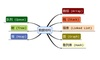数据结构 订阅